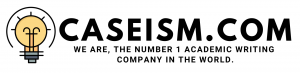# Now you see it, now you do not: the case of jet airways and its accounting policies Case Solution & Answer

## Now you see it, now you do not: the case of jet airways and its accounting policies Case Solution

### Question-1

Depreciation directly affects the value of the asset, which also reflects on the income statement as well. There is an inverse relationship between deprecation and net profit; the higher the deprecation the lower the net profit.That’s why the company has changed its policy and has decided to use straight line method of depreciation, because assets are completely written off. In addition to this,the total deprecation is also known for the assets and depreciation is the amount that you have to charge every year and subtract it from the net asset value.Therefore, it is easy to forecast the net value in future after the deprecation, for example: if the value of car is 500000 and if we sell it then its value would not be same 500000 and that is where depreciation is applicable. (Ronald Ma, 1964)

### Question-2

The similarity between reducing balance and straight-line method is that they both are based on time instead of the asset’s usage. While there is a difference which is that in straight line depreciation; the deprecation will be same throughout all the years, i.e. if the deprecation for the first year is 5000; it will be 5000 for the proceeding years as well. But, in reducing balance method; the depreciation always declines every year.

### Question – 3

Rules based deprecation principles have broad and precise elaborations concerning what is or on the other hand, isn’t permitted. These precise guidelines hold the Benefit of expanded comparability between financial reports, and make better opportunities for auditors to verify the information. (L. S. Porwal)

### Question–4

Depreciation is the amount that you have to charge every year and subtract it from the net asset value; therefore, it is easy to forecast the net value in future after the deprecation. For example: if the value of car is 100000 and if we sell then its value would not be same 100000 that is where depreciation is applicable as it would give the exact amount of depreciation every year. Deprecation is an expense that would decrease the net profit of the company butifit changes the depreciation method from reducing to straight line method, only then the deprecation would be same for the 3 years. But in reducing balance method it is more suitable because it decreases with each passing year by an otherwise strategy.  (Charles W. Lamden, 1989)

### Question – 5

From the year 2008 to 2009; there was an increase in cost and a decrease in demand. Therefore the management had to change the policy from changing its deprecation method from straight line to reducing balance method.

Reason:

• The company wanted to run its operations smoothly and in accordance with the business practice.
• There was an increase in the cost and decrease in demand, which is why the company decided to change the deprecation method to reducing balance method, through which the depreciate amount could be less every year……………………..
• This is just a sample partial case solution. Please place the order on the website to order your own originally done case solution.